# Highlight overlapping dates

This post will guide you how to find overlapping date ranges in excel, then highlight the overlapping dates. How do I highlight overlapping dates with conditional formatting function in excel.

## Highlight overlapping dates

If you want to highlight overlapping dates, you need to find the overlapping date ranges firstly, so you need to create a formula based on SUMPRODUCT function, then type this formula into the conditional formatting format box. Just do the following steps:

#1 select the cells of range that you want to find the overlapping dates (it should contain start dates and end dates)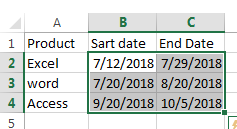#2 go to HOME tab, click Conditional Formatting command under Styles group, and select New Rule… from the drop-down menu list. The New Formatting Rule dialog will appear.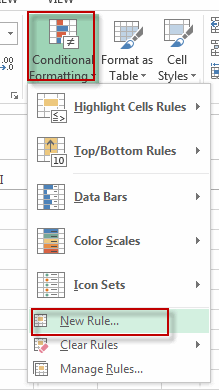#3 select Use a formula to determine which cells to format in the Select a Rule Type section, type the following formula into the Format values where this formula is true textbox.

`=SUMPRODUCT((\$B2<=\$C\$2:\$C\$4)*(\$C2>=\$B\$2:\$B\$4))>1`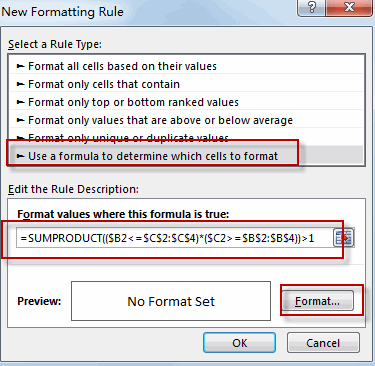#4 click Format… button, the Format cells dialog will appear. And switch to Fill tab, pick a color to be used to highlight overlapping dates. Click OK.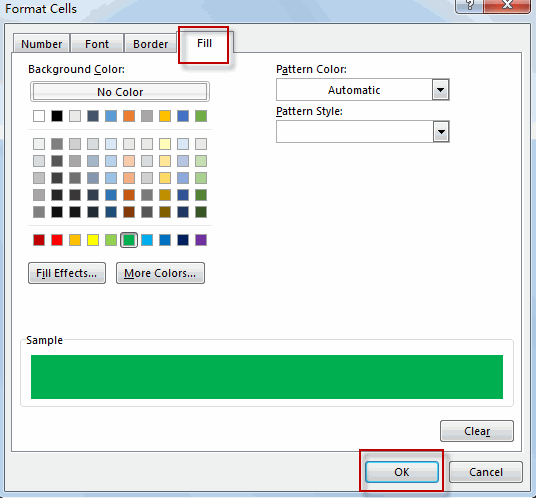#5 Click OK. You will see that all overlapping dates have been highlighted.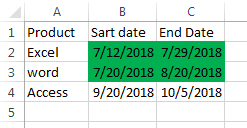If you want to highlight duplicate values and overlapping dates in excel, you just need to repeat the above steps to achieve the result. And the only different is that you need to use the following formula in the step 3:

`=SUMPRODUCT((\$B2<=\$C\$2:\$C\$5)*(\$C2>=\$B\$2:\$B\$5)*(\$A2=\$A\$2:\$A\$5))>1`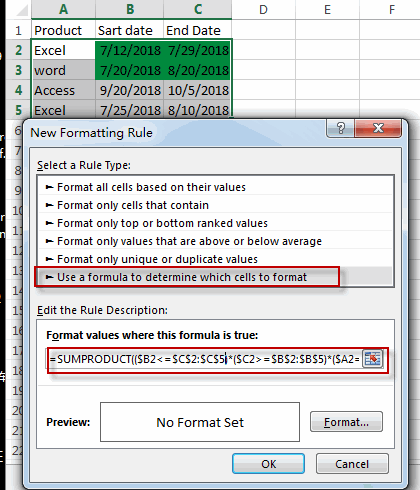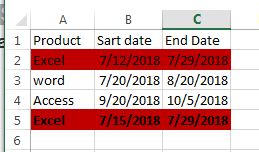### Related Functions

• Excel SUMPRODUCT function
The Excel SUMPRODUCT function multiplies corresponding components in the given one or more arrays or ranges, and returns the sum of those products.The syntax of the SUMPRODUCT function is as below:= SUMPRODUCT (array1,[array2],…)…